# ANSWER PROBLEM 3 ONLY. Here are the following links from the screenshot : https://www.geeksforgeeks.org/selection-sort/ https://www.geeksforgeeks.org/quick-sort/ https://www.geeksforgeeks.org/merge-so...ANSWER PROBLEM 3 ONLY. Here are the following links from the screenshot :

https://www.geeksforgeeks.org/selection-sort/

https://www.geeksforgeeks.org/quick-sort/

https://www.geeksforgeeks.org/merge-sort/

Problem 1 For the following list A 16, 9, 0, 4, 2, 8, 0, 0, 8, 5, 2, 1, 3, 20, 1 l,write a small program that performs the following tasks 1. Finds the mean, median and mode (without using in-built functions) Can this list have more than 1 mode? If so, your algorithm/small program should account for that, no? 2. Finds the minimum and maximum value of the list (without using in-built functions) 3. Sorts the list via bubble sort (ascending, e.g., 1, 2, 3.) 4. Sorts the list via insertion sort (ascending, e.g., 1, 2, 3..) Problem 2 For the same list as above, sort the list in such a way where the first set of numbers are ascending even and the second set of numbers are ascending odd. Your output should look like the following NOTE: Do not use the append function in Python. Try to actually write the function/algorithm to do this yourself! This is a class where you are supposed to be developing algorithms, not using in-built algorithms/ Problem 3 Sorting Extraordinaire! This problem is merely an exercise in applying some different types of sorting that the book hasn't exposed you to. Given the list above, use the following sorting types to sort the list. Sort the list two ways: Ascending & Descending 1. Selection Sort https://www.geeksforgeeks.org/selectio 2. Quick sort. w.geeksforgeeks.org/quick 3. Merge sort s://www.geeksforgeeks.org/merge-sort/ The last two algorithms are called divide & conquer algorithms which, I'm sure you can guess, means that you achieve the task in question by dividing up the list and solving it from there. They're useful, elegant and used extensively in interview questions.

1) 1(mean)1) 1 (median)1) 1 (mode)1) 21) 31) 4Similar Homework Help Questions
• ### 8. (5 points) Trace merge sort algorithm as it sorts the following sequence of integer array into...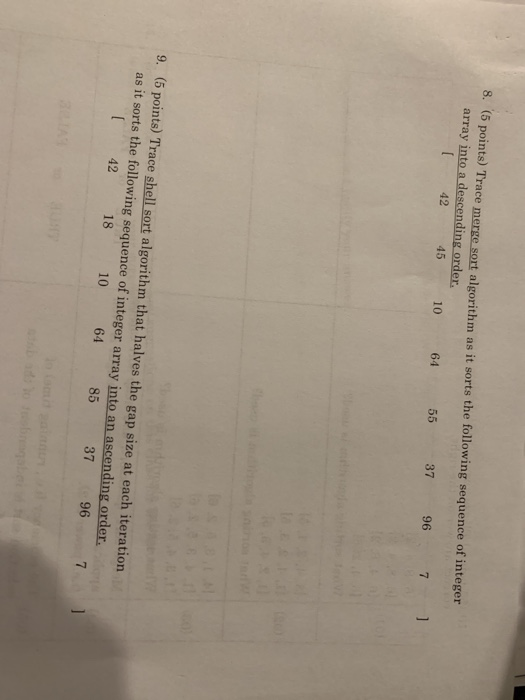8. (5 points) Trace merge sort algorithm as it sorts the following sequence of integer array into a descending order. 42 45 10 64 55 37 96 7 9. (5 points) Trace shell sort algorithm that halves the gap size at each iteration as it sorts the following sequence of integer array into an ascending order. 42 18 10 64 85 37 96 71 8. (5 points) Trace merge sort algorithm as it sorts the following sequence of integer array...

• ### 2.1 Searching and Sorting- 5 points each 1. Run Heapsort on the following array: A (7,3, 9, 4, 2,5, 6, 1,8) 2. Run merge sort on the same array. 3. What is the worst case for quick sort? What is...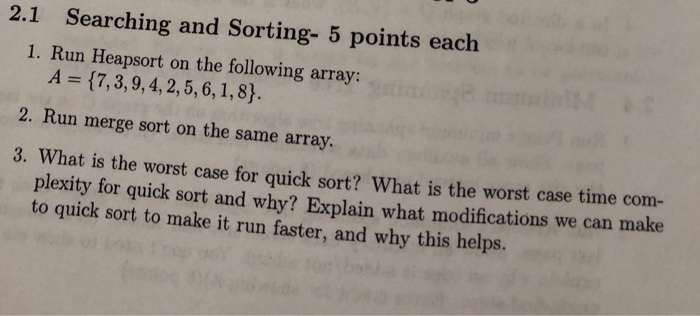2.1 Searching and Sorting- 5 points each 1. Run Heapsort on the following array: A (7,3, 9, 4, 2,5, 6, 1,8) 2. Run merge sort on the same array. 3. What is the worst case for quick sort? What is the worst case time com- plexity for quick sort and why? Explain what modifications we can make to quick sort to make it run faster, and why this helps. 4. Gi pseudocode for an algorithm that will solve the following...

• ### There are 100 integer numbers in range [1,100]. You are supposed to sort the numb...continues

There are 100 integer numbers in range [1,100]. You are supposed to sort the numbers in ascending order. E.g. 1,3,4,7,11,23, ......We know that there are dozen of sorting algorithms, such as quick sort, merge sort, bubble sort, counting sort, heap sort etc. But you have to use the followingprocedure:Step 1: Find the smallest number and its indis in the original array namely A[].Step 2: Add the smallest number to a new array namely B[].Step 3: Change the value of the...

• ### Part 1: Extend your sorting framework with two advanced sorting methods of your choice: (Shell Sort OR Radix Sort) AND (...

Part 1: Extend your sorting framework with two advanced sorting methods of your choice: (Shell Sort OR Radix Sort) AND (Merge Sort OR Quick Sort). If you choose Shell Sort, experiment with different incremental sequences to see how they affect the algorithm's run time efficiency (count the number of comparisons and exchanges). If you choose to implement Radix Sort, answer the following question as well: Can you write a version of Radix Sort to work with real numbers? If yes,...

• ### Subject: Algorithm need this urgent please. 2.1 Searching and Sorting- 5 points each 1. Run Heapsort on the following array: A 17, 3, 9, 4, 2,5, 6, 1,8) 2. Run merge sort on the same array 3. What...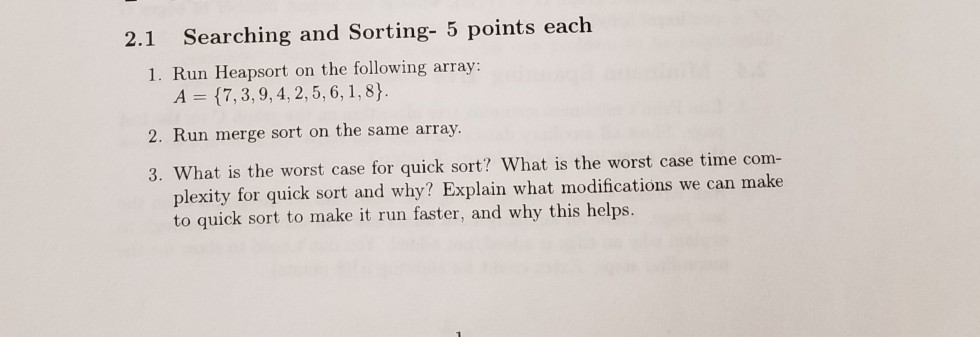Subject: Algorithm need this urgent please. 2.1 Searching and Sorting- 5 points each 1. Run Heapsort on the following array: A 17, 3, 9, 4, 2,5, 6, 1,8) 2. Run merge sort on the same array 3. What is the worst case for quick sort? What is the worst case time com- plexity for quick sort and why? Explain what modifications we can make to quick sort to make it run faster, and why this helps. 2.1 Searching and Sorting-...

• ### Here is the code given for this problem: **And please do this in Python** def mergesort(mlist): if len(mlist)<2: ret...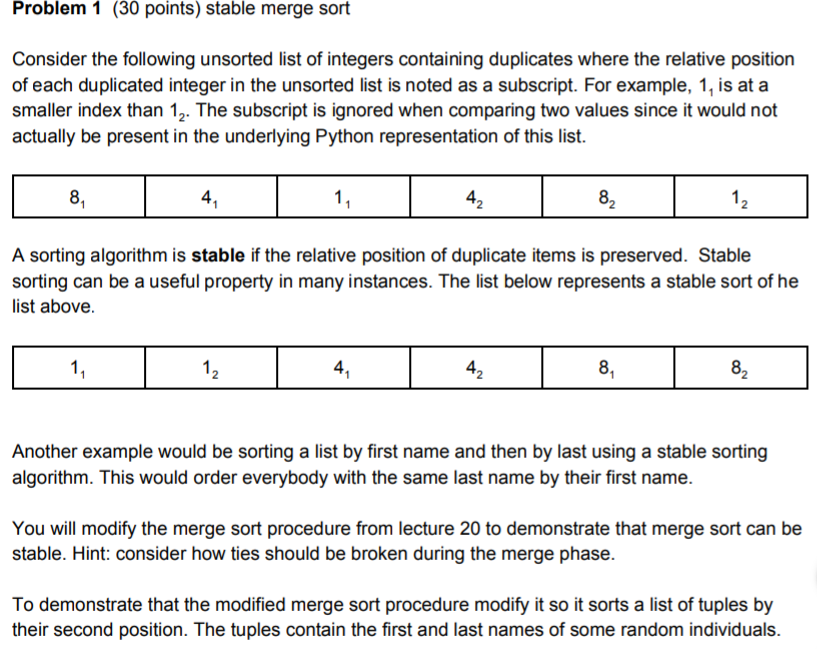Here is the code given for this problem: **And please do this in Python** def mergesort(mlist): if len(mlist)<2: return mlist else: mid=len(mlist)//2 return merge(mergesort(mlist[:mid]),mergesort(mlist[mid:])) Problem 1 (30 points) stable merge sort Consider the following unsorted list of integers containing duplicates where the relative position of each duplicated integer in the unsorted list is noted as a subscript. For example, 1, is at a smaller index than 12. The subscript is ignored when comparing two values since it would not actually...

• ### Modify the sorts (selection sort, insertion sort, bubble sort, quick sort, and merge sort) by adding code to each to tally the total number of comparisons and total execution time of each algorithm. E...

Modify the sorts (selection sort, insertion sort, bubble sort, quick sort, and merge sort) by adding code to each to tally the total number of comparisons and total execution time of each algorithm. Execute the sort algorithms against the same list, recording information for the total number of comparisons and total execution time for each algorithm. Try several different lists, including at least one that is already in sorted order. ---------------------------------------------------------------------------------------------------------------- /** * Sorting demonstrates sorting and searching on an...

• ### please I need it urgent thanks algorithms 2.1 Searching and Sorting- 5 points each 3. What is the worst case for quick sort? What is the worst case time com- plexity for quick sort and why? Ex...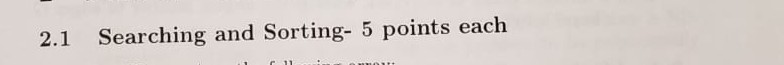please I need it urgent thanks algorithms 2.1 Searching and Sorting- 5 points each 3. What is the worst case for quick sort? What is the worst case time com- plexity for quick sort and why? Explain what modifications we can make to quick sort to make it run faster, and why this helps. 4. Give pseudocode for an algorithm that will solve the following problem. Given an array AlL..n) that contains every number between 1 and n +1 in...

• ### 1. Given the following list 107, 22, 53, 85, 21, 6, 97, 6, 32 Use merge sort to fill the followin...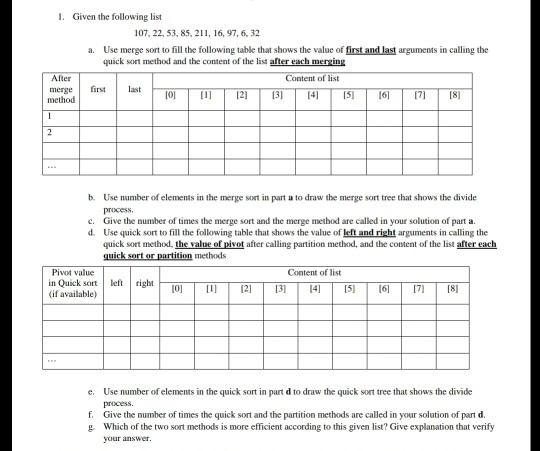1. Given the following list 107, 22, 53, 85, 21, 6, 97, 6, 32 Use merge sort to fill the following table that shows the value of first and last arguments in calling the quick sort method and the content of the list after each merging a. After f list merge firstlast method O 34 6 7 [8) b. Use number of elements in the merge sort in part a to draw the merge sort tree that shows the divide...

• ### Puodace a char showing the number of moves required to solve the Towers of Hanoi puzle using t 30...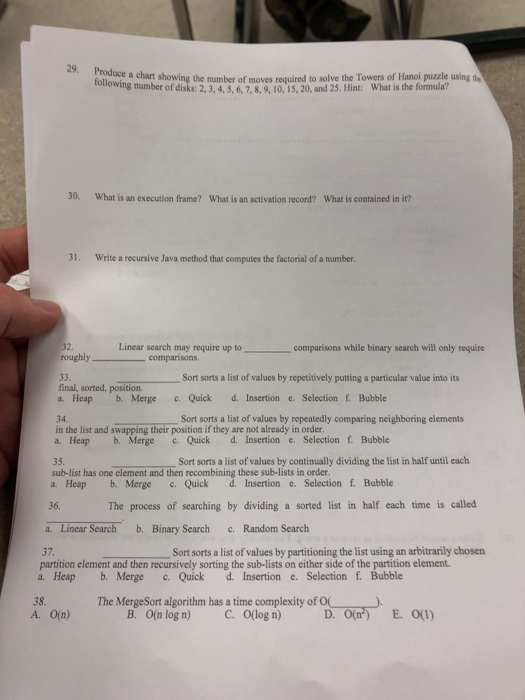Puodace a char showing the number of moves required to solve the Towers of Hanoi puzle using t 30. What is an execution frame? What is an activation record? What is contained in it? 31. Write a recursive Java method that computes the factorial of a number 2. Linear search may require up to comparisons while binary search will only require roughly comparisons 33. Sort sorts a list of values by repetitively putting a particular value into its final, sorted,...

Need Online Homework Help?